1591432405

# Convolutional Neural Network Tutorial (CNN) | How CNN Works

This Convolutional neural network tutorial (CNN) will help you understand what is a convolutional neural network, how CNN recognizes images, what are layers in the convolutional neural network and at the end, you will see a use case implementation using CNN. CNN is a feed forward neural network that is generally used to analyze visual images by processing data with grid like topology. A CNN is also known as a “ConvNet”. Convolutional networks can also perform optical character recognition to digitize text and make natural-language processing possible on analog and hand-written documents. CNNs can also be applied to sound when it is represented visually as a spectrogram. Now, lets deep dive into this video to understand what is CNN and how do they actually work.

Below topics are explained in this CNN tutorial (Convolutional Neural Network Tutorial)

1. Introduction to CNN
2. What is a convolutional neural network?
3. How CNN recognizes images?
4. Layers in convolutional neural network
5. Use case implementation using CNN

#artificial-intelligence #machine-learning

## Buddha Community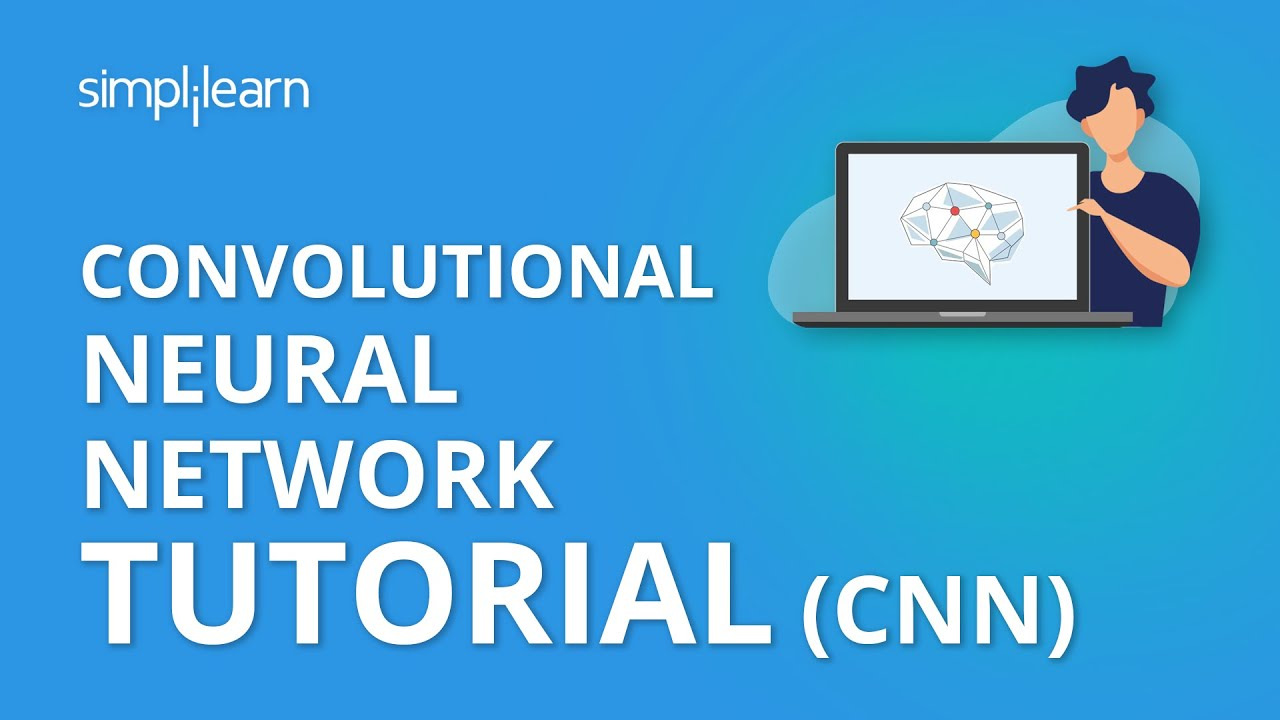1602983880

## Convolutional Neural Networks (CNN) Model from scratch for Object Detection

If you can’t explain it simply, you don’t understand it well enough - Einstein, the Man and His Achievement By G. J. Whitrow, Dover Press 1973.

CNN Model made from scratch, using the most popular Kaggle dataset  Fruits-360 and obtaining 98% accuracy.

Step 1- Importing Dataset From Kaggle to Google Colab

``````!pip install -q kaggle
``````
• Directly import the whole dataset to google colab and unzip the same
``````! mkdir ~/.kaggle
! cp kaggle.json ~/.kaggle/
! chmod 600 ~/.kaggle/kaggle.json

! mkdir fruits
! unzip fruits.zip -d fruits
``````

#image-recognition #cnn #data-science #convolutional-neural-net #neural-networks1594274580

## CNN Series Part 1: How do computers see images?

In this article, we will learn about how computers see images & the issues faced while performing a computer vision task. We will see how deep learning comes into the picture & how with the power of neural networks, we can build a powerful computer vision system capable of solving extraordinary problems.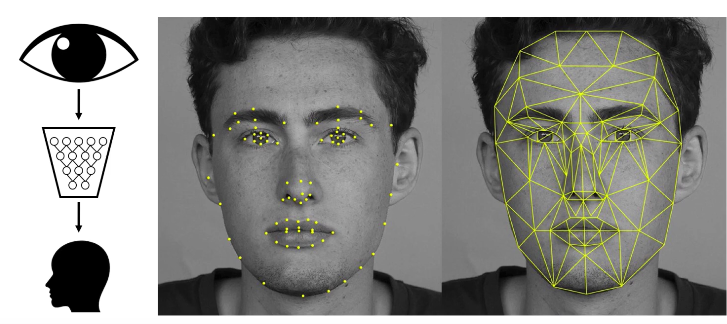One example of how deep learning is transforming computer vision is facial recognition or face detection. On the top left, you can see the icon of the human eye which visually represents vision coming into the deep neural network in the form of images, pixels, videos & on the output on the bottom you can see a depiction of the human face or detection of the human face or this could also be recognizing different human faces or emotions on the face and also the key facial features, etc.

#convolution-neural-net #computer-vision #neural-networks #cnn #convolutional-network #series1599214860

## Convolutional Neural Networks, Explained

A Convolutional Neural Network, also known as CNN or ConvNet, is a class of neural networks that specializes in processing data that has a grid-like topology, such as an image. A digital image is a binary representation of visual data. It contains a series of pixels arranged in a grid-like fashion that contains pixel values to denote how bright and what color each pixel should be.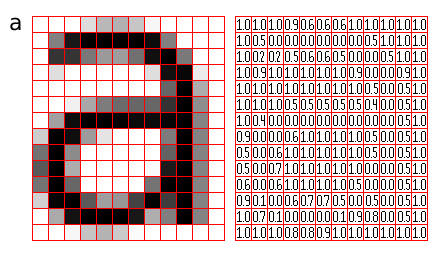Figure 1: Representation of image as a grid of pixels (Source)

The human brain processes a huge amount of information the second we see an image. Each neuron works in its own receptive field and is connected to other neurons in a way that they cover the entire visual field. Just as each neuron responds to stimuli only in the restricted region of the visual field called the receptive field in the biological vision system, each neuron in a CNN processes data only in its receptive field as well. The layers are arranged in such a way so that they detect simpler patterns first (lines, curves, etc.) and more complex patterns (faces, objects, etc.) further along. By using a CNN, one can enable sight to computers.

## Convolutional Neural Network Architecture

A CNN typically has three layers: a convolutional layer, a pooling layer, and a fully connected layer.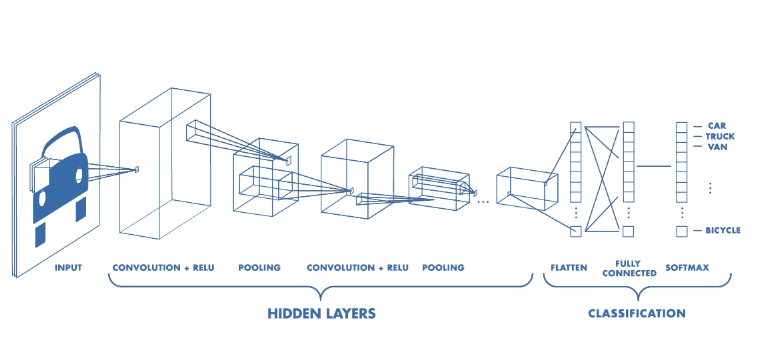Figure 2: Architecture of a CNN (Source)

## Convolution Layer

The convolution layer is the core building block of the CNN. It carries the main portion of the network’s computational load.

This layer performs a dot product between two matrices, where one matrix is the set of learnable parameters otherwise known as a kernel, and the other matrix is the restricted portion of the receptive field. The kernel is spatially smaller than an image but is more in-depth. This means that, if the image is composed of three (RGB) channels, the kernel height and width will be spatially small, but the depth extends up to all three channels.

#computer-vision #writing-nn #convolution-network #cnn #fashion-mnist #neural networks1595691960

## How Graph Convolutional Networks (GCN) Work

In this post, we’re gonna take a close look at one of the well-known Graph neural networks named GCN. First, we’ll get the intuition to see how it works, then we’ll go deeper into the maths behind it.

## Why Graphs?

Many problems are graphs in true nature. In our world, we see many data are graphs, such as molecules, social networks, and paper citations networks.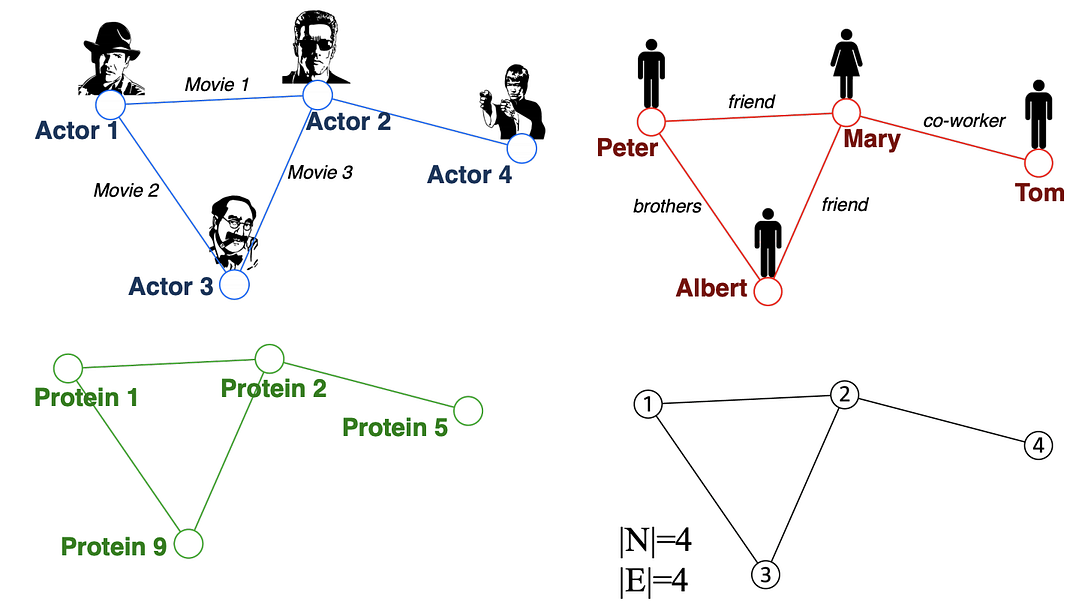Examples of graphs. (Picture from )

• Node classification: Predict a type of a given node
• Community detection: Identify densely linked clusters of nodes
• Network similarity: How similar are two (sub)networks

## Machine Learning Lifecycle

In the graph, we have node features (the data of nodes) and the structure of the graph (how nodes are connected).

For the former, we can easily get the data from each node. But when it comes to the structure, it is not trivial to extract useful information from it. For example, if 2 nodes are close to one another, should we treat them differently to other pairs? How about high and low degree nodes? In fact, each specific task can consume a lot of time and effort just for Feature Engineering, i.e., to distill the structure into our features.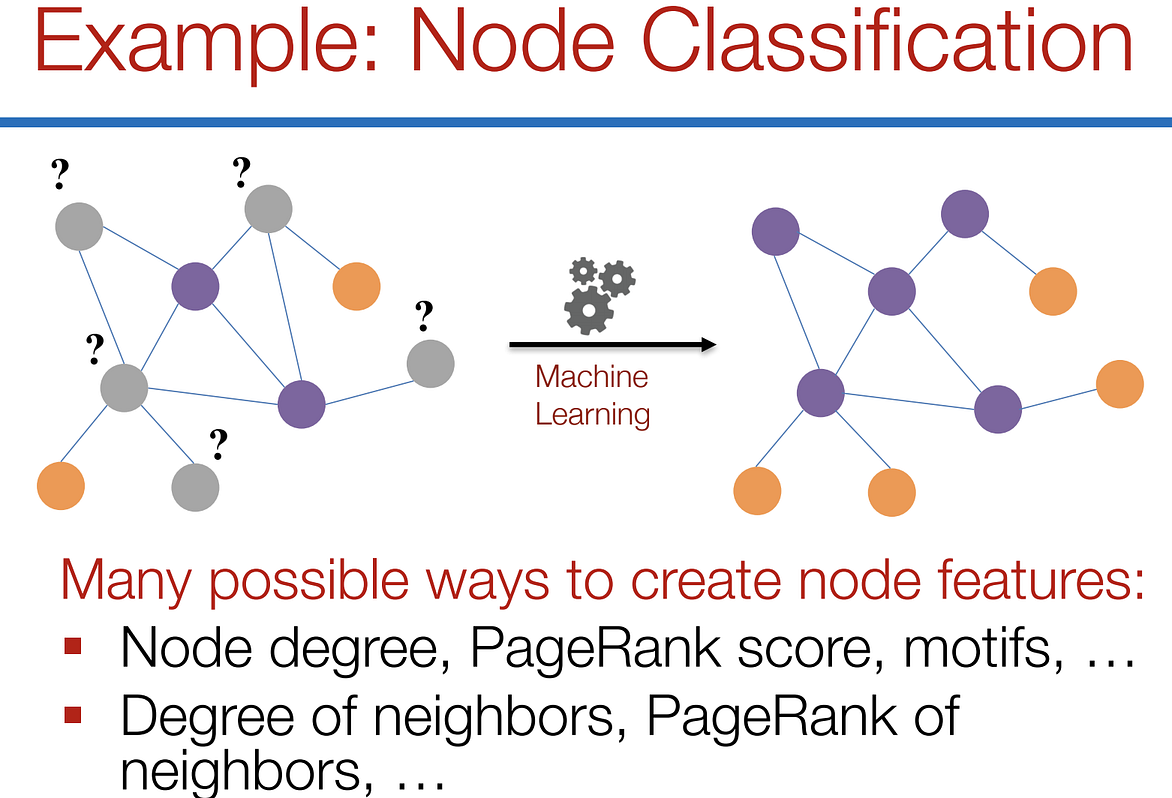Feature engineering on graphs. (Picture from )

It would be much better to somehow get both the node features and the structure as the input, and let the machine to figure out what information is useful by itself.

That’s why we need Graph Representation Learning.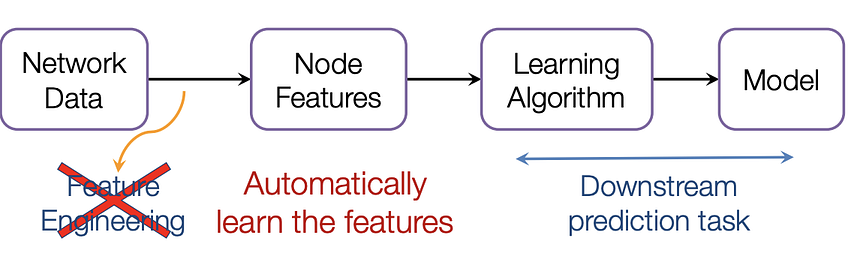We want the graph can learn the “feature engineering” by itself. (Picture from )

# Graph Convolutional Networks (GCNs)

Paper: Semi-supervised Classification with Graph Convolutional Networks(2017) 

GCN is a type of convolutional neural network that can work directly on graphs and take advantage of their structural information.

it solves the problem of classifying nodes (such as documents) in a graph (such as a citation network), where labels are only available for a small subset of nodes (semi-supervised learning).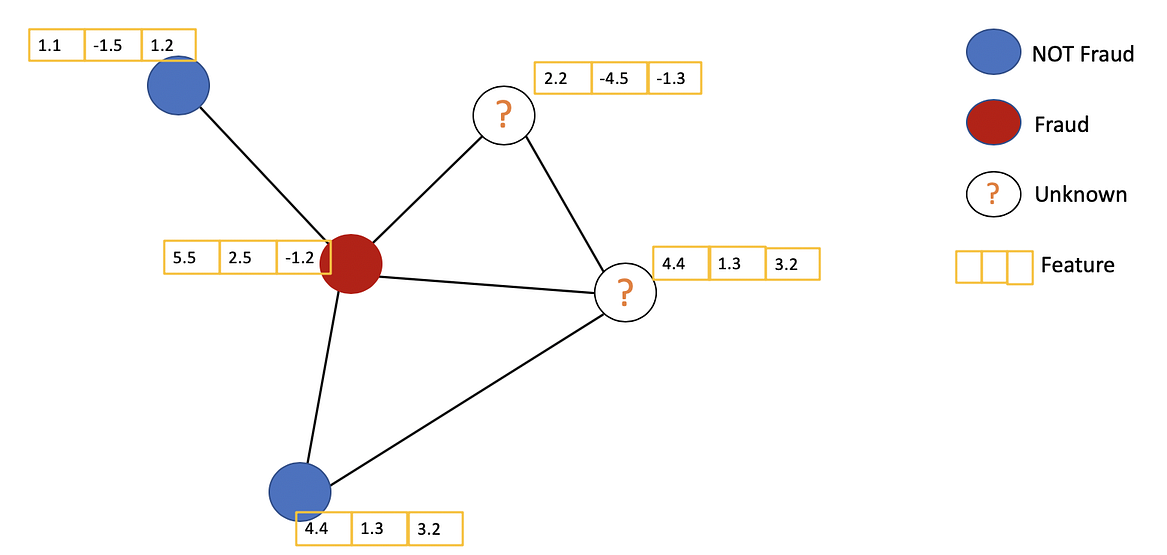Example of Semi-supervised learning on Graphs. Some nodes dont have labels (unknown nodes).

#graph-neural-networks #graph-convolution-network #deep-learning #neural-networks1604977629

## 10 Free Online Resources To Learn Convolutional Neural Networks

C onvolutional Neural Networks (CNNs) are one of the most important neural network algorithms in the present scenario. Tech giants like Google, Facebook, Amazon have been thoroughly using this neural network to perform and achieve a number of image-related tasks.

The  applications of CNNs mostly includes the field of computer vision for image recognition, object detection, among others, This neural network is also being used for video inputs, speech recognition, sentence modelling, etc. in NLP models and more.

Below, we have curated a list of 10 best free online resources, in no particular order, to learn  Convolutional Neural Networks (CNNs).

#### Convolutional Neural Networks

About: This course is a part of the Deep Learning Specialisation at Coursera. Here, you will learn how to build  convolutional neural networks and apply them to image data. You will understand how to build a CNN model, understand the recent variations, know how to apply  convolutional networks to visual detection as well as recognition tasks and more.

Know more here.

#### Introducing Convolutional Neural Networks

**About: **This tutorial is curated by the developers at Google. This tutorial, encompasses a brief introduction on  convolution neural networks (CNNs), how it works, including hands-on training. You will learn topics like ReLU, pooling, fully connected layers and more.

Know more here.

#### Convolution Neural Networks for Visual Recognition

**About: **This is a free course where you will learn about  convolution neural networks and how they can be used in visual recognition. The tutorial starts with an architecture overview and then moves into ConvNet layers such as normalisation layer, fully connected layer, etc. including its architectures, such as layer patterns, computational considerations and more.

Know more here.

#cnn neural network #cnns #convolutional neural networks